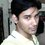# May Lord ... [Backstage]

You must be familiar with the formulas:

$$\sin { A/2 } +\cos { A/2 } =\pm \sqrt { 1+\sin { A } }$$

$\sin { A/2 } -\cos { A/2 } =\pm \sqrt { 1-\sin { A } }$

The Problem May Lord... is related to problems such as expressing trigonometrical ratios of angle A/2 in terms of $\sin { A }$.

There is always an ambiguity in such cases.

What I am trying to say is that knowing the value of $\sin { A }$ does not uniquely determine the value of $\sin { A/2 }$ and $\cos { A/2 }$ but only gives the magnitude or absolute value of $\sin { A/2 }$ and $\cos { A/2 }$.To obtain the trigonometrical ratios completely,of A/2,in terms of $\sin { A }$ we also need to find its sign [ +,-]. But,to determine the sign [ +,-] we need to know the quadrant in which the angle lies.

To find the ambiguities we can proceed d as follows:

$\sin { A/2 } +\cos { A/2 }$

$=\sqrt { 2 } \left( \frac { 1 }{ \sqrt { 2 } } \sin { A/2 } +\frac { 1 }{ \sqrt { 2 } } \cos { A/2 } \right)$

$=\sqrt { 2 } \sin { (\pi /4+A/2 } )\quad$ ...(i)

(i) is positive if$A/2+\pi /4$ lies between $2n\pi$ and $2n\pi +\pi$

i.e A/2 lies between $2n\pi -\pi /4$ and $2n\pi +3\pi /4$

$\therefore$ $\sin { A/2 } +\cos { A/2 }$ is positive if

A/2 lies between $2n\pi -\pi /4$ and $2n\pi +3\pi /4$

It is negative otherwise. [i.e between $2n\pi +3\pi /4$ and $2n\pi+7\pi /4$ ]

In just similar way it can be shown that $sin { A/2 } -cos { A/2 }$ is positive

if A/2 lies between $2n\pi +\pi /4$ and $2n\pi +5\pi /4$.

It is negative otherwise. [i.e between $2n\pi -3\pi /4$ and $2n\pi +\pi /4$ ]

It will be much better to understand if you have a diagram[I have a diagram but I don't know how to upload it here,sorry for that].Draw the renowned four quadrants then mark of $\pi /4,3\pi /4,5\pi /4,7\pi /4$.

This is all you require to shoot May Lord forgive us our sins

Some Examples:

• Q# In the formula $2\cos { A/2 } =\pm \sqrt { 1+\sin { A } } \pm \sqrt { 1-\sin { A } }$

Find within what limits A\2 must lie when:

1.the two positive signs are taken

2.the two negative signs are taken

solution: 1. The formula $2\cos { A/2 } =+\sqrt { 1+\sin { A } } +\sqrt { 1-\sin { A } }$

Is obtained using the following two formulas:

$\sin { A/2 } +\cos { A/2 } =+\sqrt { 1+\sin { A } } \\ \sin { A/2 } -\cos { A/2 } =-\sqrt { 1-\sin { A } }$

Now $\sin { A/2 } +\cos { A/2 }$ is +ve between $2n\pi -\pi /4$ and $2n\pi +3\pi /4$ and $\sin { A/2 } -\cos { A/2 }$ is -ve between $2n\pi -3\pi /4$ and $2n\pi +\pi /4$.Take the intersection.

Hence A/2 must lie within$2n\pi -\pi /4$ and $2n\pi +\pi /4$.

2.The formula $2\cos { A/2 } =-\sqrt { 1+\sin { A } } -\sqrt { 1-\sin { A } }$

Is obtained using the following two formulas:

$sin { A/2 } +\cos { A/2 } =-\sqrt { 1+\sin { A } } \\ \sin { A/2 } -\cos { A/2 } =+\sqrt { 1-\sin { A } }$

Now $\sin { A/2 } +\cos { A/2 }$ is -ve between $2n\pi+3\pi /4$ and $2n\pi+7\pi /4$ and $\sin { A/2 } -\cos { A/2 }$ is +ve between $2n\pi+\pi /4$ and $2n\pi+5\pi /4$. Take the intersection.

Hence A/2 must lie within $2n\pi+3\pi /4$ and $2n\pi+5\pi /4$.Note by Soumo Mukherjee
6 years, 8 months ago

This discussion board is a place to discuss our Daily Challenges and the math and science related to those challenges. Explanations are more than just a solution — they should explain the steps and thinking strategies that you used to obtain the solution. Comments should further the discussion of math and science.

When posting on Brilliant:

• Use the emojis to react to an explanation, whether you're congratulating a job well done , or just really confused .
• Ask specific questions about the challenge or the steps in somebody's explanation. Well-posed questions can add a lot to the discussion, but posting "I don't understand!" doesn't help anyone.
• Try to contribute something new to the discussion, whether it is an extension, generalization or other idea related to the challenge.
• Stay on topic — we're all here to learn more about math and science, not to hear about your favorite get-rich-quick scheme or current world events.

MarkdownAppears as
*italics* or _italics_ italics
**bold** or __bold__ bold
- bulleted- list
• bulleted
• list
1. numbered2. list
1. numbered
2. list
Note: you must add a full line of space before and after lists for them to show up correctly
paragraph 1paragraph 2

paragraph 1

paragraph 2

[example link](https://brilliant.org)example link
> This is a quote
This is a quote
    # I indented these lines
# 4 spaces, and now they show
# up as a code block.

print "hello world"
# I indented these lines
# 4 spaces, and now they show
# up as a code block.

print "hello world"
MathAppears as
Remember to wrap math in $$ ... $$ or $ ... $ to ensure proper formatting.
2 \times 3 $2 \times 3$
2^{34} $2^{34}$
a_{i-1} $a_{i-1}$
\frac{2}{3} $\frac{2}{3}$
\sqrt{2} $\sqrt{2}$
\sum_{i=1}^3 $\sum_{i=1}^3$
\sin \theta $\sin \theta$
\boxed{123} $\boxed{123}$

## Comments

There are no comments in this discussion.

×

Problem Loading...

Note Loading...

Set Loading...